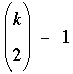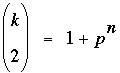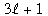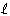Math Central - mathcentral.uregina.caProblem of the MonthCurrentproblem Recent problemswith solutions
 Older problems from 2005/2006 06/07 07/08 08/09 09/10 10/11 11/12

Solution for November 2008

 The Problem:MP81 November 2008 For which k ≥ 3 is aprime power pn?

Correct responses:Our problem is Problem 984 in Crux Mathematicorum with Mathematical Mayhem, 12:1 (January 1986), pages 15-16 (in the days when the journal was just Crux Math).   Solutions were submitted to us by

 Bojan Basic (Serbia) Farid Alberto Lian Martínez (Colombia) Gérad Billion (France) Matthew Lim (USA) Jean Braconnier (France) Sébastien Racanière (UK) Olivier Cyr (France) John T. Robinson (USA) Dan Dima (Romania) Albert Stadler (Switzerland) Philippe Fondanaiche (France) Bernard Carpentier (France) Catherine-A. Nadault (France)

however two of our correspondents were a bit careless this month and overlooked a couple possibilities, so their solutions were incomplete.

The solution:

Solution.  k can take the values 3, 4, 5, or 8.  All of the submitted solutions came down to a similar argument.  Letwhere k and n are positive integers, p prime.  Then we are given

(k – 2)(k + 1) = 2pn,                                                        (1)

so that either (i) k – 2 is even or (ii) k + 1 is even.

Case (i).  Let k – 2 = 2m, m ≥ 1.  Then (1) becomes m(2m + 3) = pn, which implies m = pr and

2pr + 3 = pn–r.                                                                   (2)

1. r = 0.  By (2) we have m = 1 and 2m + 3 = 5 = pn; thus, k = 4, pn = 5.

2. r ≥ 1.  Now p must divide both sides of (2), whence p divides 3 and, therefore, p = 3.  Equation (2) is then 2·3r + 3 = 3n–r, from which we get 2·3r–1 + 1 = 3n-r–1.  The right-hand side cannot be 1 (because that would imply 2·3r–1 = 0); thus r – 1 = 0 (otherwise the left-hand side would befor some nonzero integer, which would not be divisible by 3).  We conclude that n = 3, m = 3, and 2m + 3 = 9, implying for this subcase that k = 8, pn = 27.

Case (ii).  Let k + 1 = 2m, m > 1.  Then (1) becomes m(2m – 3) = pn, which implies m = pr for some r ≥ 1, and

2pr – 3 = pn–r.                                                                   (3)

1. n – r = 0.  By (3) we have r = n = 1 and p = 2; thus, k = 3, pn = 2.

2. n – r ≥ 1.  Divisibility arguments applied to (3) again imply that p = 3, whence equation (3) becomes 2·3r–1 – 1 = 3n-r–1.  Again either 3r–1 or 3n-r–1 must be 1.  Here these conditions are equivalent giving us r = 1 and n = 2, and therefore,
k = 5, pn = 9.

 Math Central is supported by the University of Regina and the Pacific Institute for the Mathematical Sciences.about math central :: site map :: links :: notre site français